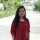All About Software Testing In One Place!
124 Members
Join this group to post and comment.Computer Science
14 Jan 2019

# What is equivalence class partition?

Explain?Computer Science
2mos ago

Equivalence class partition (ECP)is a testing technique. The idea behind this technique is to divide a set of test conditions into groups that can be considered to be same.

One test value is picked from each class while testing. This is because we are assuming that all the conditions in one partition will be treated in the same way by the software.Each and every condition of particular partition works same as other. If a condition in a partition works, other conditions work too. If a condition doesn't work or is invalid, other conditions are invalid too.

Advantage of ECP -Less number of test cases

It reduces the time required for performing testing of a software due to less number of test cases.

Example

Suppose we have to test a field that accepts only teenagers

Valid input:13– 19

Invalid input:less than 13(<13), greater than 19 (>19)

Valid Class: 13– 19  Pick any one input test data from 13 – 19

Invalid Class : <13 Any input test data less than 13

Invalid Class : >19 Any input test data greater than 19

So,all possible test cases have been categorized into 3 classes.Shruthi Pillai
Information Technology
2mos ago

It is a black box technique .You divide the set of test condition into a partition  .You can apply this technique, where there is a range in the input field.

FOR EG:

We have a order pizza textbox.

PIzza from 1 to 10 are considered as valid.

11 to 99 as invalid. if any number given in this range, error message should be shown. A user can order only max 10 pizzas.

SO THE TEST CONDITIONS ARE

1.Any number greater than 10 is invalid.

2.Any number less than 1 ie,0 will be invalid.

3.Numbers 1 to 10 will be valid.

4.Any 3 digit number will be invalid.

We cannot test all the values. we divide possible values into groups or sets. The divided sets are called equivalence partitions or classes.In this technique, if any one value of partition pass, all others will be pass. IF any value fails, all other values in the partition considered as fail